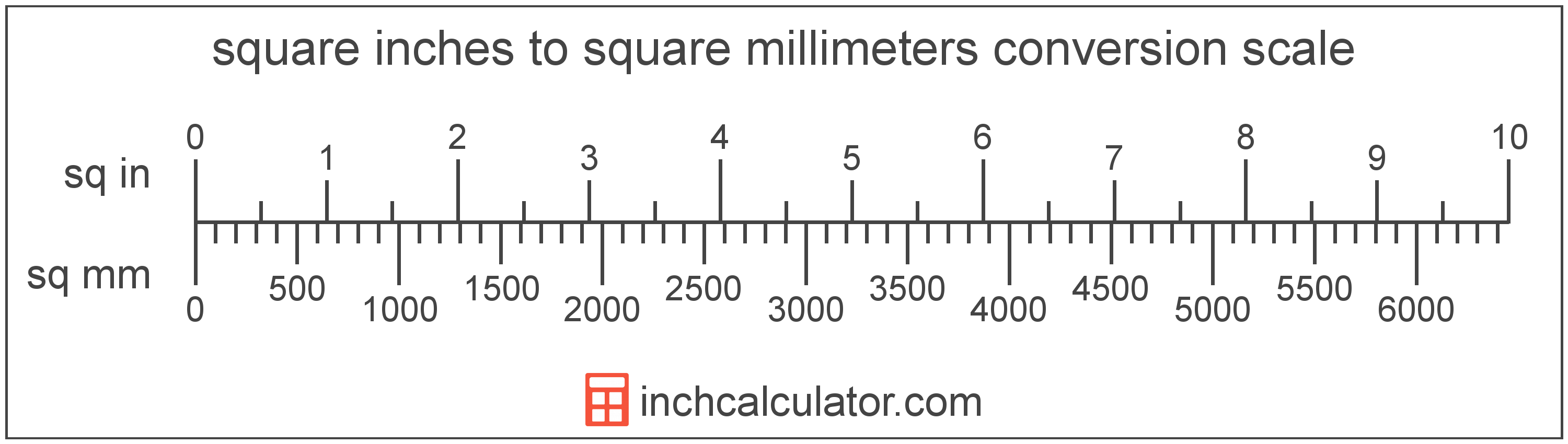# Square Inches to Square Millimeters Converter

Enter the area in square inches below to get the value converted to square millimeters.

Results in Square Millimeters:1 sq in = 645.16 sq mm
1 sq in = 6.4516 sq cm

Do you want to convert square millimeters to square inches?

## How to Convert Square Inches to Square Millimeters

To convert a measurement in square inches to a measurement in square millimeters, multiply the area by the following conversion ratio: 645.16 square millimeters/square inch.

Since one square inch is equal to 645.16 square millimeters, you can use this simple formula to convert:

square millimeters = square inches × 645.16

The area in square millimeters is equal to the area in square inches multiplied by 645.16.

For example, here's how to convert 5 square inches to square millimeters using the formula above.
square millimeters = (5 sq in × 645.16) = 3,225.8 sq mm### How Many Square Millimeters Are in a Square Inch?

There are 645.16 square millimeters in a square inch, which is why we use this value in the formula above.

1 sq in = 645.16 sq mm

## What is a Square Inch?

One square inch is equivalent to the area of a square with sides that are each 1 inch in length. One square inch is equal to 1/144 of a square foot or 1/1,296 of a square yard.

The square inch is a US customary and imperial unit of area. A square inch is sometimes also referred to as a square in. Square inches can be abbreviated as sq in, and are also sometimes abbreviated as in². For example, 1 square inch can be written as 1 sq in or 1 in².

## What is a Square Millimeter?

One square millimeter is equivalent to the area of a square with edges that are each 1 millimeter in length. One square millimeter is equal to 0.00155 square inches or 1/100 of a square centimeter.

The square millimeter, or square millimetre, is a multiple of the square meter, which is the SI derived unit for area. In the metric system, "milli" is the prefix for thousandths, or 10-3. A square millimeter is sometimes also referred to as a square mm. Square millimeters can be abbreviated as sq mm, and are also sometimes abbreviated as mm². For example, 1 square millimeter can be written as 1 sq mm or 1 mm².

## Square Inch to Square Millimeter Conversion Table

Table showing various square inch measurements converted to square millimeters.
Square Inches Square Millimeters
1 sq in 645.16 sq mm
2 sq in 1,290 sq mm
3 sq in 1,935 sq mm
4 sq in 2,581 sq mm
5 sq in 3,226 sq mm
6 sq in 3,871 sq mm
7 sq in 4,516 sq mm
8 sq in 5,161 sq mm
9 sq in 5,806 sq mm
10 sq in 6,452 sq mm
11 sq in 7,097 sq mm
12 sq in 7,742 sq mm
13 sq in 8,387 sq mm
14 sq in 9,032 sq mm
15 sq in 9,677 sq mm
16 sq in 10,323 sq mm
17 sq in 10,968 sq mm
18 sq in 11,613 sq mm
19 sq in 12,258 sq mm
20 sq in 12,903 sq mm
21 sq in 13,548 sq mm
22 sq in 14,194 sq mm
23 sq in 14,839 sq mm
24 sq in 15,484 sq mm
25 sq in 16,129 sq mm
26 sq in 16,774 sq mm
27 sq in 17,419 sq mm
28 sq in 18,064 sq mm
29 sq in 18,710 sq mm
30 sq in 19,355 sq mm
31 sq in 20,000 sq mm
32 sq in 20,645 sq mm
33 sq in 21,290 sq mm
34 sq in 21,935 sq mm
35 sq in 22,581 sq mm
36 sq in 23,226 sq mm
37 sq in 23,871 sq mm
38 sq in 24,516 sq mm
39 sq in 25,161 sq mm
40 sq in 25,806 sq mm